This book is archived and will be removed July 6, 2022. Please use the updated version.

Particle Physics and Cosmology

# 84 Particle Accelerators and Detectors

### Learning Objectives

By the end of this section, you will be able to:

• Compare and contrast different types of particle accelerators
• Describe the purpose, components, and function of a typical colliding beam machine
• Explain the role of each type of subdetector of a typical multipurpose particle detector
• Use the curvature of a charge track to determine the momentum of a particle

The goal of experimental particle physics is to accurately measure elementary particles. The primary method used to achieve this end is to produce these particles in high-energy collisions and then measure the products of using highly sensitive particle detectors. These experiments are used to test and revise scientific models of particle interactions. The purpose of this section is to describe particle accelerators and detectors. Modern machines are based on earlier ones, so it is helpful to present a brief history of accelerators and detectors.

### Early Particle Accelerators

A particle accelerator is a machine designed to accelerate charged particles. This acceleration is usually achieved with strong electric fields, magnetic fields, or both. A simple example of a particle accelerator is the Van de Graaff accelerator (see Electric Potential). This type of accelerator collects charges on a hollow metal sphere using a moving belt. When the electrostatic potential difference of the sphere is sufficiently large, the field is used to accelerate particles through an evacuated tube. Energies produced by a Van de Graaff accelerator are not large enough to create new particles, but the machine was important for early exploration of the atomic nucleus.

Larger energies can be produced by a linear accelerator (called a “linac”). Charged particles produced at the beginning of the linac are accelerated by a continuous line of charged hollow tubes. The voltage between a given pair of tubes is set to draw the charged particle in, and once the particle arrives, the voltage between the next pair of tubes is set to push the charged particle out. In other words, voltages are applied in such a way that the tubes deliver a series of carefully synchronized electric kicks ((Figure)). Modern linacs employ radio frequency (RF) cavities that set up oscillating electromagnetic fields, which propel the particle forward like a surfer on an ocean wave. Linacs can accelerate electrons to over 100 MeV. (Electrons with kinetic energies greater than 2 MeV are moving very close to the speed of light.) In modern particle research, linear accelerators are often used in the first stage of acceleration.

In a linear accelerator, charged tubes accelerate particles in a series of electromagnetic kicks. Each tube is longer than the preceding tube because the particle is moving faster as it accelerates.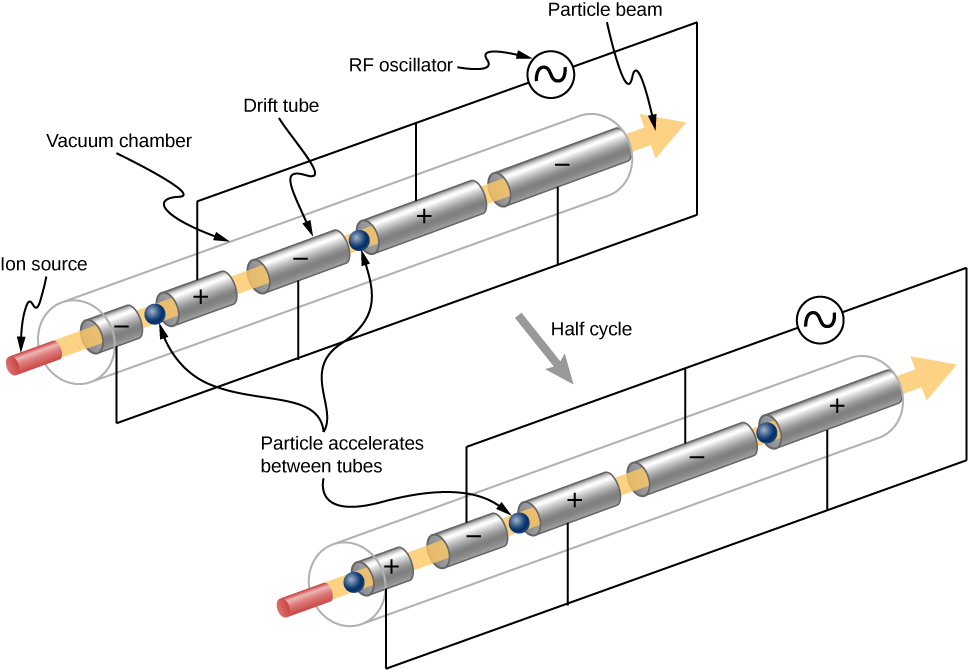Accelerating Tubes A linear accelerator designed to produce a beam of 800-MeV protons has 2000 accelerating tubes separated by gaps. What average voltage must be applied between tubes to achieve the desired energy? (Hint: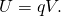Strategy The energy given to the proton in each gap between tubes is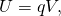where q is the proton’s charge and V is the potential difference (voltage) across the gap. Since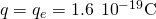and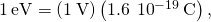the proton gains 1 eV in energy for each volt across the gap that it passes through. The ac voltage applied to the tubes is timed so that it adds to the energy in each gap. The effective voltage is the sum of the gap voltages and equals 800 MV to give each proton an energy of 800 MeV.

Solution There are 2000 gaps and the sum of the voltages across them is 800 MV. Therefore, the average voltage applied is 0.4 MV or 400 kV.

Significance A voltage of this magnitude is not difficult to achieve in a vacuum. Much larger gap voltages would be required for higher energy, such as those at the 50-GeV SLAC facility. Synchrotrons are aided by the circular path of the accelerated particles, which can orbit many times, effectively multiplying the number of accelerations by the number of orbits. This makes it possible to reach energies greater than 1 TeV.

Check Your Understanding How much energy does an electron receive in accelerating through a 1-V potential difference?

1 eV

The next-generation accelerator after the linac is the cyclotron ((Figure)). A cyclotron uses alternating electric fields and fixed magnets to accelerate particles in a circular spiral path. A particle at the center of the cyclotron is first accelerated by an electric field in a gap between two D-shaped magnets (Dees). As the particle crosses over the D-shaped magnet, the particle is bent into a circular path by a Lorentz force. (The Lorentz force was discussed in Magnetic Forces and Fields.) Assuming no energy losses, the momentum of the particle is related to its radius of curvature by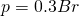where p is the momentum in GeV/c, B is in teslas, and r is the radius of the trajectory (“orbit”) in meters. This expression is valid to classical and relativistic velocities. The circular trajectory returns the particle to the electric field gap, the electric field is reversed, and the process continues. As the particle is accelerated, the radius of curvature gets larger and larger—spirally outward—until the electrons leave the device.

Cyclotrons use a magnetic field to cause particles to move in circular orbits. As the particles pass between the plates of the “Dees,” the voltage across the gap is reversed so the particles are accelerated twice in each orbit.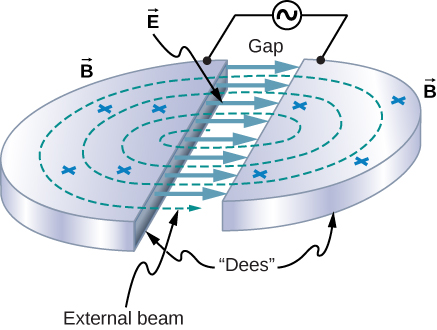A synchrotron is a circular accelerator that uses alternating voltage and increasing magnetic field strength to accelerate particles to higher energies. Charged particles are accelerated by RF cavities, and steered and focused by magnets. RF cavities are synchronized to deliver “kicks” to the particles as they pass by, hence the name. Steering high-energy particles requires strong magnetic fields, so superconducting magnets are often used to reduce heat losses. As the charged particles move in a circle, they radiate energy: According to classical theory, any charged particle that accelerates (and circular motion is an accelerated motion) also radiates. In a synchrotron, such radiation is called synchrotron radiation. This radiation is useful for many other purposes, such as medical and materials research.

The Energy of an Electron in a Cyclotron An electron is accelerated using a cyclotron. If the magnetic field is 1.5 T and the radius of the “Dees” is 1.2 m, what is the kinetic energy of the outgoing particle?

Strategy If the radius of orbit of the electron exceeds the radius of the “Dees,” the electron exits the device. So, the radius of the “Dees” places an upper limit on the radius and, therefore, the momentum and energy of the accelerated particle. The exit momentum of the particle is determined using the radius of orbit and strength of the magnetic field. The exit energy of the particle can be determined the particle momentum (Relativity).

Solution Assuming no energy losses, the momentum of the particle in the cyclotron is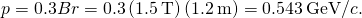The momentum energy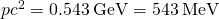is much larger than the rest mass energy of the electron,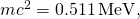so relativistic expression for the energy of the electron must be used (see Relativity). The total energy of the electron is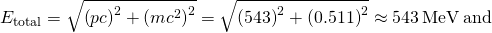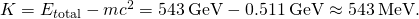Significance The total energy of the electron is much larger than its rest mass energy. In other words, the total energy of the electron is almost all in the form of kinetic energy. Cyclotrons can be used to conduct nuclear physics experiments or in particle therapy to treat cancer.

Check Your Understanding A charged particle of a certain momentum travels in an arc through a uniform magnetic field. What happens if the magnetic field is doubled?

The radius of the track is cut in half.

### Colliding Beam Machines

New particles can be created by colliding particles at high energies. According to Einstein’s mass-energy relation, the energies of the colliding particles are converted into mass energy of the created particle. The most efficient way to do this is with particle-colliding beam machines. A colliding beam machine creates two counter-rotating beams in a circular accelerator, stores the beams at constant energy, and then at the desired moment, focuses the beams on one another at the center of a sensitive detector.

The prototypical colliding beam machine is the Cornell Electron Storage Ring, located in Ithaca, New York ((Figure)). Electrons (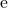) and positrons (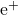) are created at the beginning of the linear accelerator and are accelerated up to 150 MeV. The particles are then injected into the inner synchrotron ring, where they are accelerated by RF cavities to 4.5 to 6 GeV. When the beams are up to speed, they are transferred and “stored” in an outer storage ring at the same energy. The two counter-rotating beams travel through the same evacuated pipe, but are kept apart until collisions are desired. The electrons and positrons circle the machine in bunches 390,000 times every second.

The Cornell Electron Storage Ring uses a linear accelerator and a synchrotron to accelerate electrons and positrons to 4.5–6 GeV. The particles are held in the outer storage ring at that energy until they are made to collide in a particle detector. (credit: modification of work by Laboratory of Nuclear Studies, Cornell Electron Storage Ring)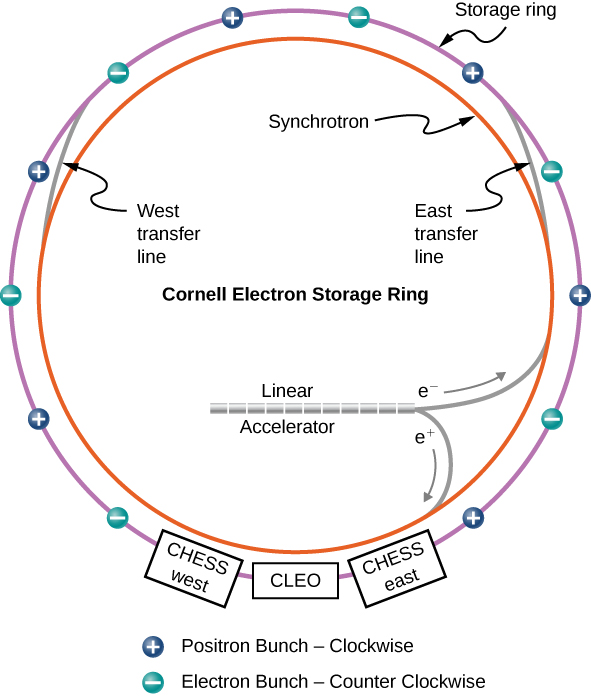When an electron and positron collide, they annihilate each other to produce a photon, which exists for too short a time to be detected. The photon produces either a lepton pair (e.g., an electron and position, muon or antimuon, or tau and antitau) or a quark pair. If quarks are produced, mesons form, such as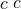and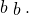These mesons are created nearly at rest since the initial total momentum of the electron-positron system is zero. Note, mesons cannot be created at just any colliding energy but only at “resonant” energies that correspond to the unique masses of the mesons ((Figure)). The mesons created in this way are highly unstable and decay quickly into lighter particles, such as electrons, protons, and photons. The collision “fragments” provide valuable information about particle interactions.

As the field of particle physics advances, colliding beam machines are becoming more powerful. The Large Hadron Collider (LHC), currently the largest accelerator in the world, collides protons at beam energies exceeding 6 TeV. The center-of-mass energy (W) refers to the total energy available to create new particles in a colliding machine, or the total energy of incoming particles in the center-of-mass frame. (The concept of a center-of-mass frame of reference is discussed in Linear Momentum and Collisions.) Therefore, the LHC is able to produce one or more particles with a total mass exceeding 12 TeV. The center-of-mass energy is given by: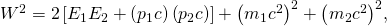where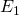and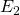are the total energies of the incoming particles (1 and 2),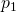and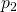are the magnitudes of their momenta, and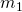and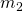are their rest masses.

Creating a New Particle The mass of the upsilon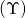meson (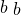) is created in a symmetric electron-positron collider. What beam energy is required?

StrategyThe Particle Data Group has stated that the rest mass energy of this meson is approximately 10.58 GeV. The above expression for the center-of-mass energy can be simplified because a symmetric collider implies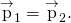Also, the rest masses of the colliding electrons and positrons are identical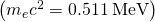and much smaller than the mass of the energy particle created. Thus, the center-of-mass energy (W) can be expressed completely in terms of the beam energy,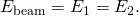Solution Based on the above assumptions, we have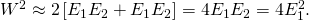The beam energy is therefore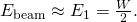The rest mass energy of the particle created in the collision is equal to the center-of-mass energy, so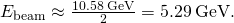Significance Given the energy scale of this problem, the rest mass energy of the upsilonmeson is due almost entirely due to the initial kinetic energies of the electron and positrons. This meson is highly unstable and quickly decays to lighter and more stable particles. The existence of the upsilonparticle appears as a dramatic increase of such events at 5.29 GeV.

Check Your Understanding Why is a symmetric collider “symmetric?”

The colliding particles have identical mass but opposite vector momenta.

Higher beam energies require larger accelerators, so modern colliding beam machines are very large. The LHC, for example, is 17 miles in circumference ((Figure)). (In the 1940s, Enrico Fermi envisioned an accelerator that encircled all of Earth!) An important scientific challenge of the twenty-first century is to reduce the size of particle accelerators.

### Particle Detectors

The purpose of a particle detector is to accurately measure the outcome of collisions created by a particle accelerator. The detectors are multipurpose. In other words, the detector is divided into many subdetectors, each designed to measure a different aspect of the collision event. For example, one detector might be designed to measure photons and another might be designed to measure muons. To illustrate how subdetectors contribute to an understanding of an entire collision event, we describe the subdetectors of the Compact Muon Solenoid (CMS), which was used to discover the Higgs Boson at the LHC ((Figure)).

Compact Muon Solenoid detector. The detector consists of several layers, each responsible for measuring different types of particles. (credit: modification of work by David Barney/CERN)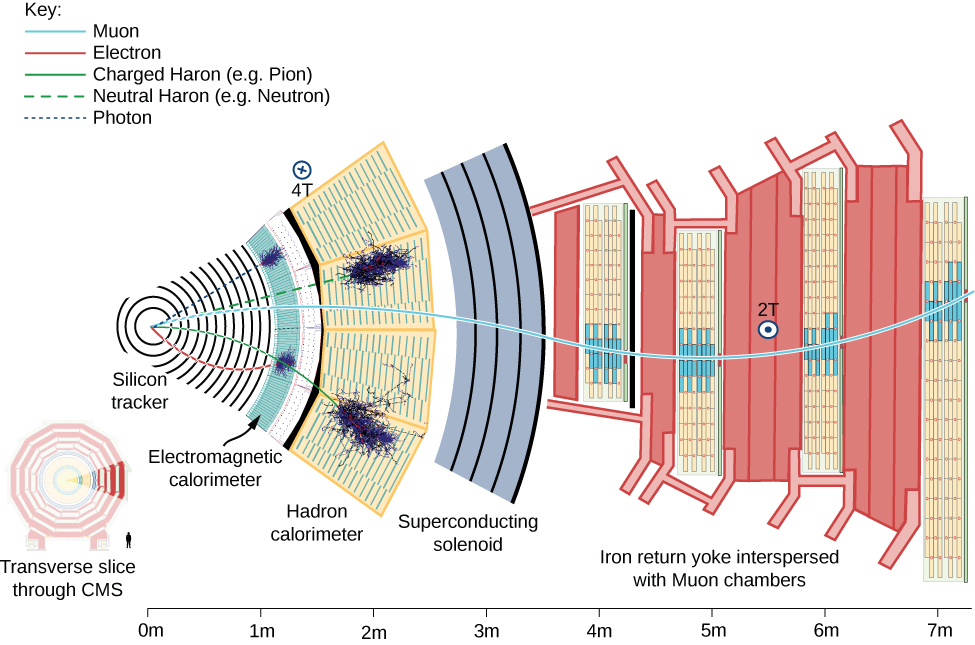The beam pipe of the detector is out of (and into) the page at the left. Particles produced by pp collisions (the “collision fragments”) stream out of the detector in all directions. These particles encounter multiple layers of subdetectors. A subdetector is a particle detector within a larger system of detectors designed to measure certain types of particles. There are several main types of subdetectors. Tracking devices determine the path and therefore momentum of a particle; calorimeters measure a particle’s energy; and particle-identification detectors determine a particle’s identity (mass).

The first set of subdetectors that particles encounter is the silicon tracking system. This system is designed to measure the momentum of charged particles (such as electrons and protons). The detector is bathed in a uniform magnetic field, so the charged particles are bent in a circular path by a Lorentz force (as for the cyclotron). If the momentum of the particle is large, the radius of the trajectory is large, and the path is almost straight. But if the momentum is small, the radius of the trajectory is small, and the path is tightly curved. As the particles pass through the detector, they interact with silicon microstrip detectors at multiple points. These detectors produce small electrical signals as the charged particles pass near the detector elements. The signals are then amplified and recorded. A series of electrical “hits” is used to determine the trajectory of the particle in the tracking system. A computer-generated “best fit” to this trajectory gives the track radius and therefore the particle momentum. At the LHC, a large number of tracks are recorded for the same collision event. Fits to the tracks are shown by the blue and green lines in (Figure).

A three-dimensional view of particle fragments in the LHC as seen by the ATLAS detector. (credit: LHC/CERN)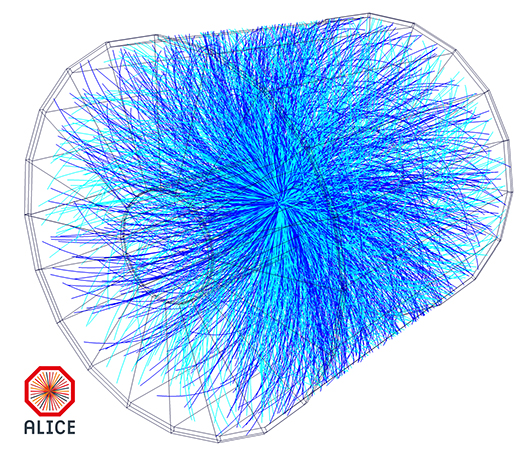Beyond the tracking layers is the electromagnetic calorimeter. This detector is made of clear, lead-based crystals. When electrons interact with the crystals, they radiate high-energy photons. The photons interact with the crystal to produce electron-positron pairs. Then, these particles radiate more photons. The process repeats, producing a particle shower (the crystal “glows”). A crude model of this process is as follows.

An electron with energy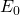strikes the crystal and loses half of its energy in the form of a photon. The photon produces an electron-positron pair, and each particle proceeds away with half the energy of the photon. Meanwhile, the original electron radiates again. So, we are left with four particles: two electrons, one positron, and one photon, each with an energy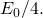The number of particles in the shower increases geometrically. After n radiation events, there are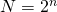particles. Hence, the total energy per particle after n radiation events is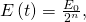whereis the incident energy and E(t) is the amount of energy per particle after n events. An incoming photon triggers a similar chain of events ((Figure)). If the energy per particle drops below a particular threshold value, other types of radiative processes become important and the particle shower ceases. Eventually, the total energy of the incoming particle is absorbed and converted into an electrical signal.

(a) A particle shower produced in a crystal calorimeter. (b) A diagram showing a typical sequence of reactions in a particle shower.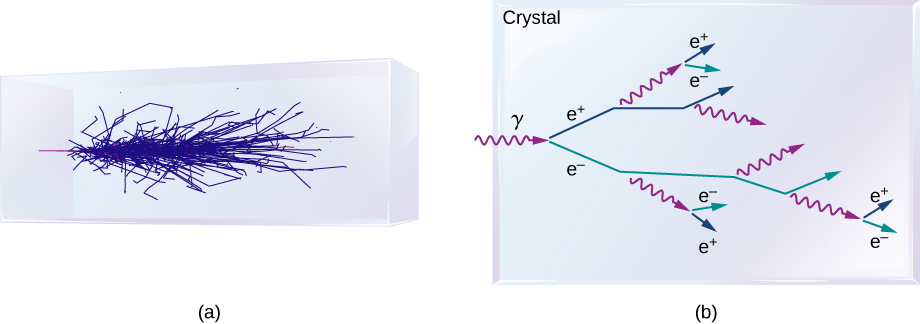Beyond the crystal calorimeter is the hadron calorimeter. As the name suggests, this subdetector measures hadrons such as protons and pions. The hadron calorimeter consists of layers of brass and steel separated by plastic scintillators. Its purpose is to absorb the particle energy and convert it into an electronic signal. Beyond this detector is a large magnetic coil used to produce a uniform field for tracking.

The last subdetector is the muon detector, which consists of slabs of iron that only muons (and neutrinos) can penetrate. Between the iron slabs are multiple types of muon-tracking elements that accurately measure the momentum of the muon. The muon detectors are important because the Higgs boson (discussed soon) can be detected through its decays to four muons—hence the name of the detector.

Once data is collected from each of the particle subdetectors, the entire collision event can be assessed. The energy of the ith particle is written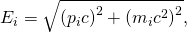where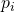is the absolute magnitude of the momentum of the ith particle, and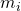is its rest mass.

The total energy of all particles is therefore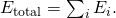If all particles are detected, the total energy should be equal to the center-of-mass energy of the colliding beam machine (W). In practice, not all particles are identified, either because these particles are too difficult to detect (neutrinos) or because these particles “slip through.” In many cases, whole chains of decays can be “reconstructed,” like putting back together a watch that has been smashed to pieces. Information about these decay chains are critical to the evaluation of models of particle interactions.

### Summary

• Many types of particle accelerators have been developed to study particles and their interactions. These include linear accelerators, cyclotrons, synchrotrons, and colliding beams.
• Colliding beam machines are used to create massive particles that decay quickly to lighter particles.
• Multipurpose detectors are used to design all aspects of high-energy collisions. These include detectors to measure the momentum and energies of charge particles and photons.
• Charged particles are measured by bending these particles in a circle by a magnetic field.
• Particles are measured using calorimeters that absorb the particles.

### Conceptual Questions

Briefly compare the Van de Graaff accelerator, linear accelerator, cyclotron, and synchrotron accelerator.

Describe the basic components and function of a typical colliding beam machine.

the “linac” to accelerate the particles in a straight line, a synchrotron to accelerate and store the moving particles in a circular ring, and a detector to measure the products of the collisions

What are the subdetectors of the Compact Muon Solenoid experiment? Briefly describe them.

What is the advantage of a colliding-beam accelerator over one that fires particles into a fixed target?

In a colliding beam experiment, the energy of the colliding particles goes into the rest mass energy of the new particle. In a fix-target experiment, some of this energy is lost to the momentum of the new particle since the center-of-mass of colliding particles is not fixed.

An electron appears in the muon detectors of the CMS. How is this possible?

### Problems

A charged particle in a 2.0-T magnetic field is bent in a circle of radius 75 cm. What is the momentum of the particle?

A proton track passes through a magnetic field with radius of 50 cm. The magnetic field strength is 1.5 T. What is the total energy of the proton?

965 GeV

Derive the equationusing the concepts of centripetal acceleration (Motion in Two and Three Dimensions) and relativistic momentum (Relativity)

Assume that beam energy of an electron-positron collider is approximately 4.73 GeV. What is the total mass (W) of a particle produced in the annihilation of an electron and positron in this collider? What meson might be produced?

According to (Figure),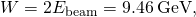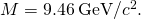This is the mass of the upsilon (1S) meson first observed at Fermi lab in 1977. The upsilon meson consists of a bottom quark and its antiparticle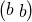.

At full energy, protons in the 2.00-km-diameter Fermilab synchrotron travel at nearly the speed of light, since their energy is about 1000 times their rest mass energy. (a) How long does it take for a proton to complete one trip around? (b) How many times per second will it pass through the target area?

Suppose a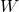created in a particle detector lives for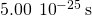. What distance does it move in this time if it is traveling at 0.900c? (Note that the time is longer than the givenlifetime, which can be due to the statistical nature of decay or time dilation.)

0.135 fm; Since this distance is too short to make a track, the presence of themust be inferred from its decay products.

What length track does a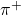traveling at 0.100c leave in a bubble chamber if it is created there and lives for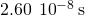? (Those moving faster or living longer may escape the detector before decaying.)

The 3.20-km-long SLAC produces a beam of 50.0-GeV electrons. If there are 15,000 accelerating tubes, what average voltage must be across the gaps between them to achieve this energy?

3.33 MV

### Glossary

particle accelerator
machine designed to accelerate charged particles; this acceleration is usually achieved with strong electric fields, magnetic fields, or both
particle detector
detector designed to accurately measure the outcome of collisions created by a particle accelerator; particle detectors are hermetic and multipurpose
synchrotron
circular accelerator that uses alternating voltage and increasing magnetic field strengths to accelerate particles to higher and higher energies